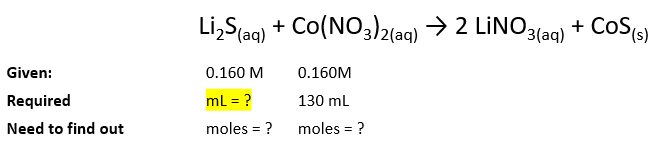# Problem: Consider the following reaction: Li2S(aq) + Co(NO3)2(aq) → 2 LiNO3(aq) + CoS(s)What volume of 0.160 M Li2S solution is required to completely react with 130 mL of 0.160 M Co(NO3)2?

###### FREE Expert Solution

So, we will begin solving this problem by analyzing what information we have, to solve this problem:We will by doing these steps:

1. Finding the moles of 0.160 M Co(NO3)2 from given molarity and volume
2. Finding the moles of Li2S from the moles of Co(NO3)2 using the molar ratio
3. Finding the volume of Li2S using its molarity.

Step 1

Recall that:###### Problem Details

Consider the following reaction: Li2S(aq) + Co(NO3)2(aq) → 2 LiNO3(aq) + CoS(s)

What volume of 0.160 M Li2S solution is required to completely react with 130 mL of 0.160 M Co(NO3)2?